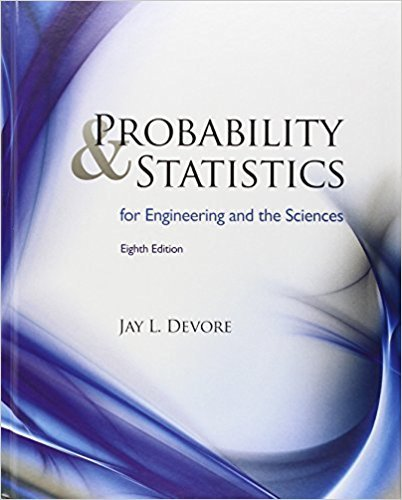×
×

# Solutions for Chapter 12: Simple Linear Regression and Correlation## Full solutions for Probability and Statistics for Engineering and the Sciences | 8th Edition

ISBN: 9780538733526Solutions for Chapter 12: Simple Linear Regression and Correlation

Solutions for Chapter 12
4 5 0 389 Reviews
16
3
##### ISBN: 9780538733526

This expansive textbook survival guide covers the following chapters and their solutions. Chapter 12: Simple Linear Regression and Correlation includes 87 full step-by-step solutions. Since 87 problems in chapter 12: Simple Linear Regression and Correlation have been answered, more than 28887 students have viewed full step-by-step solutions from this chapter. This textbook survival guide was created for the textbook: Probability and Statistics for Engineering and the Sciences , edition: 8. Probability and Statistics for Engineering and the Sciences was written by and is associated to the ISBN: 9780538733526.

Key Statistics Terms and definitions covered in this textbook

A variation of the R 2 statistic that compensates for the number of parameters in a regression model. Essentially, the adjustment is a penalty for increasing the number of parameters in the model. Alias. In a fractional factorial experiment when certain factor effects cannot be estimated uniquely, they are said to be aliased.

• Bayes’ estimator

An estimator for a parameter obtained from a Bayesian method that uses a prior distribution for the parameter along with the conditional distribution of the data given the parameter to obtain the posterior distribution of the parameter. The estimator is obtained from the posterior distribution.

• Bayes’ theorem

An equation for a conditional probability such as PA B ( | ) in terms of the reverse conditional probability PB A ( | ).

• Biased estimator

Unbiased estimator.

• Bimodal distribution.

A distribution with two modes

• Cause-and-effect diagram

A chart used to organize the various potential causes of a problem. Also called a ishbone diagram.

• Completely randomized design (or experiment)

A type of experimental design in which the treatments or design factors are assigned to the experimental units in a random manner. In designed experiments, a completely randomized design results from running all of the treatment combinations in random order.

• Conditional probability density function

The probability density function of the conditional probability distribution of a continuous random variable.

• Conditional variance.

The variance of the conditional probability distribution of a random variable.

• Critical region

In hypothesis testing, this is the portion of the sample space of a test statistic that will lead to rejection of the null hypothesis.

• Curvilinear regression

An expression sometimes used for nonlinear regression models or polynomial regression models.

• Dispersion

The amount of variability exhibited by data

• Distribution function

Another name for a cumulative distribution function.

• Estimator (or point estimator)

A procedure for producing an estimate of a parameter of interest. An estimator is usually a function of only sample data values, and when these data values are available, it results in an estimate of the parameter of interest.

• Exhaustive

A property of a collection of events that indicates that their union equals the sample space.

• Exponential random variable

A series of tests in which changes are made to the system under study

• Finite population correction factor

A term in the formula for the variance of a hypergeometric random variable.

• Fraction defective control chart

See P chart

• Generator

Effects in a fractional factorial experiment that are used to construct the experimental tests used in the experiment. The generators also deine the aliases.

• Geometric mean.

The geometric mean of a set of n positive data values is the nth root of the product of the data values; that is, g x i n i n = ( ) = / w 1 1 .

×